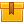# Transformers

## Prerequisites

The lesson requires a fundamental knowledge of electrical theory and technology. Completion of the lesson, Introduction to Transformers, is required.

## Description

This is the final lesson in the iKNOW Electrical Theory for Troubleshooters Library. This lesson builds on the information presented in the lesson, Introduction to Transformers.
How to determine primary current and voltage, secondary current and voltage, and load is taught. The function of various transformers is also explained.

## Objectives

Given the secondary voltage and load, determine the primary current. Given the primary voltage and load, determine the secondary current. Given the turns ratio and voltage and current from either the primary or secondary, determine the power. Given power and primary voltage, determine primary current. Given primary voltage, determine secondary voltage in a 3-phase transformer. Describe the configuration of a 3-phase transformer. State some applications and maintenance precautions for a 3-phase transformer. Explain the functions and uses of multi-tap transformer and auto-transformers.

## ContentThis is the final lesson in the iKNOW™ Electrical Theory for
Troubleshooters Library. This lesson builds on the information presented
in the lesson, Introduction to Transformers.  How to determine primary current and voltage, secondary current and
voltage, and load is taught. The function of various transformers is
also explained.

•ETFT Transfomers

0
0 Reviews